# Describing Data Frequency Tables Frequency Distributions and Graphic

• Slides: 21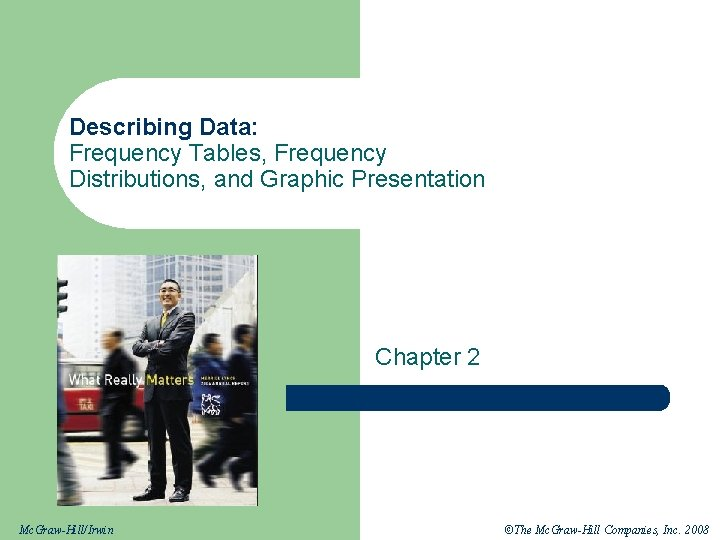Describing Data: Frequency Tables, Frequency Distributions, and Graphic Presentation Chapter 2 Mc. Graw-Hill/Irwin ©The Mc. Graw-Hill Companies, Inc. 2008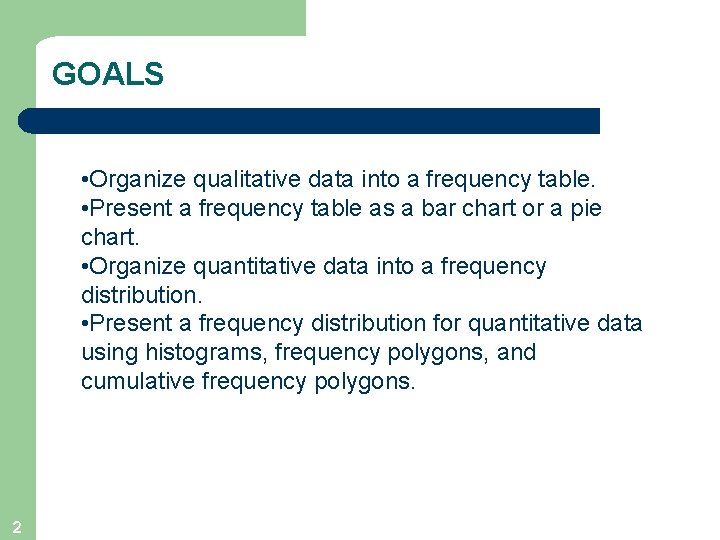GOALS • Organize qualitative data into a frequency table. • Present a frequency table as a bar chart or a pie chart. • Organize quantitative data into a frequency distribution. • Present a frequency distribution for quantitative data using histograms, frequency polygons, and cumulative frequency polygons. 2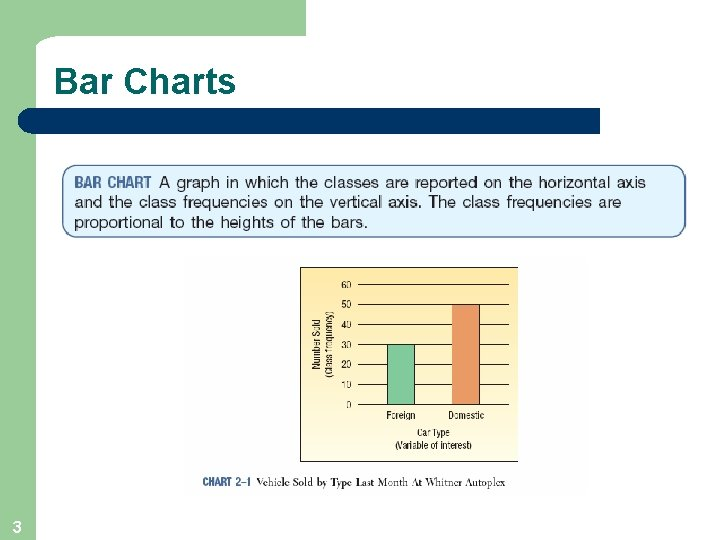Bar Charts 3Pie Charts 4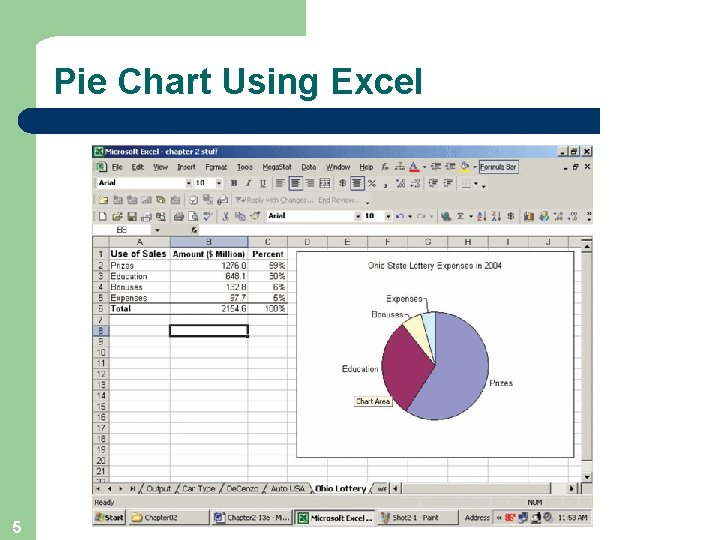Pie Chart Using Excel 5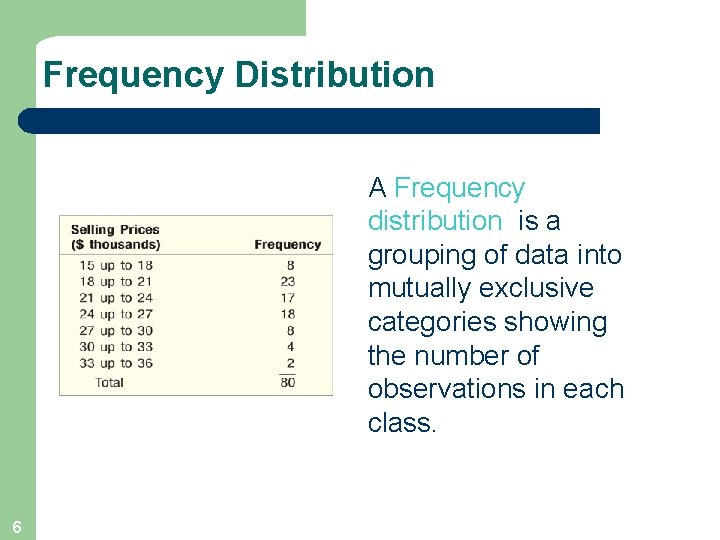Frequency Distribution A Frequency distribution is a grouping of data into mutually exclusive categories showing the number of observations in each class. 6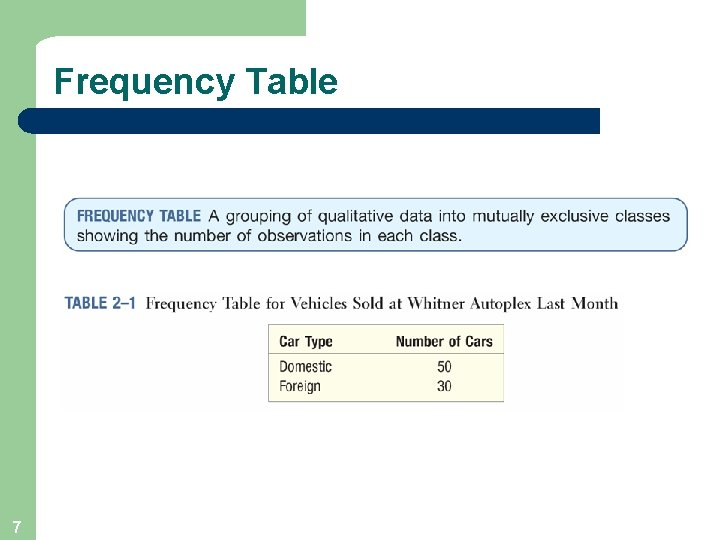Frequency Table 7Relative Class Frequencies l l 8 Class frequencies can be converted to relative class frequencies to show the fraction of the total number of observations in each class. A relative frequency captures the relationship between a class total and the total number of observations.Frequency Distribution Class midpoint: A point that divides a class into two equal parts. This is the average of the upper and lower class limits. Class frequency: The number of observations in each class. Class interval: The class interval is obtained by subtracting the lower limit of a class from the lower limit of the next class. 9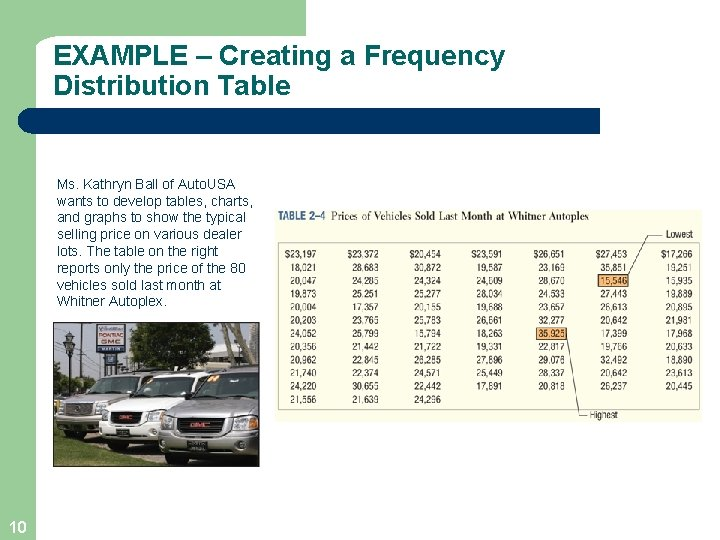EXAMPLE – Creating a Frequency Distribution Table Ms. Kathryn Ball of Auto. USA wants to develop tables, charts, and graphs to show the typical selling price on various dealer lots. The table on the right reports only the price of the 80 vehicles sold last month at Whitner Autoplex. 10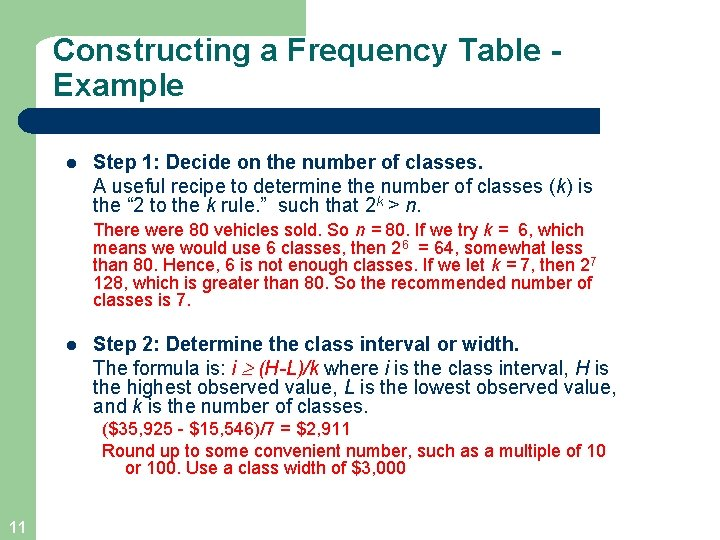Constructing a Frequency Table Example l Step 1: Decide on the number of classes. A useful recipe to determine the number of classes (k) is the “ 2 to the k rule. ” such that 2 k > n. There were 80 vehicles sold. So n = 80. If we try k = 6, which means we would use 6 classes, then 26 = 64, somewhat less than 80. Hence, 6 is not enough classes. If we let k = 7, then 27 128, which is greater than 80. So the recommended number of classes is 7. l Step 2: Determine the class interval or width. The formula is: i (H-L)/k where i is the class interval, H is the highest observed value, L is the lowest observed value, and k is the number of classes. (\$35, 925 - \$15, 546)/7 = \$2, 911 Round up to some convenient number, such as a multiple of 10 or 100. Use a class width of \$3, 000 11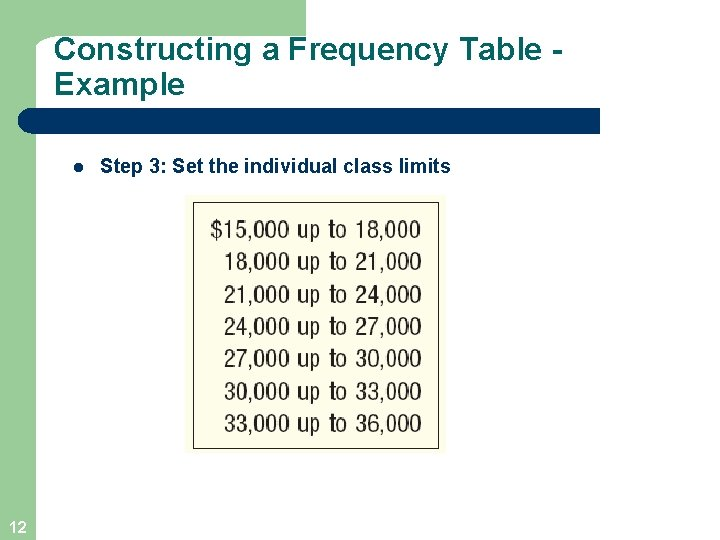Constructing a Frequency Table Example l 12 Step 3: Set the individual class limits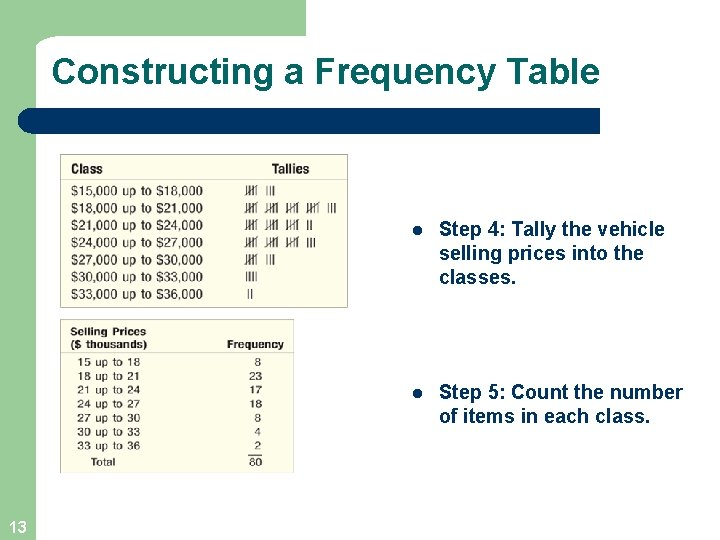Constructing a Frequency Table 13 l Step 4: Tally the vehicle selling prices into the classes. l Step 5: Count the number of items in each class.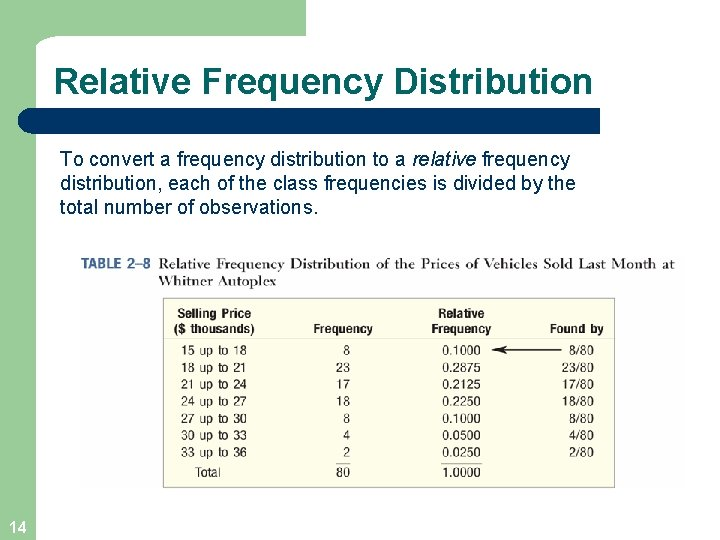Relative Frequency Distribution To convert a frequency distribution to a relative frequency distribution, each of the class frequencies is divided by the total number of observations. 14Graphic Presentation of a Frequency Distribution The three commonly used graphic forms are: l Histograms l Frequency polygons l Cumulative frequency distributions 15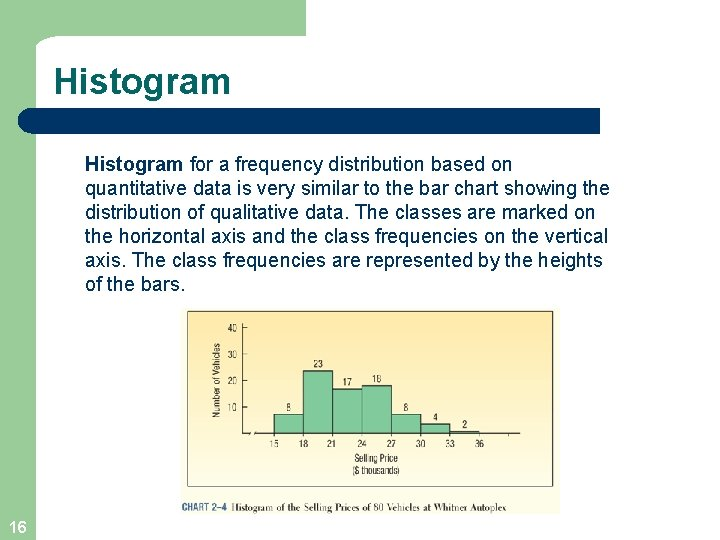Histogram for a frequency distribution based on quantitative data is very similar to the bar chart showing the distribution of qualitative data. The classes are marked on the horizontal axis and the class frequencies on the vertical axis. The class frequencies are represented by the heights of the bars. 16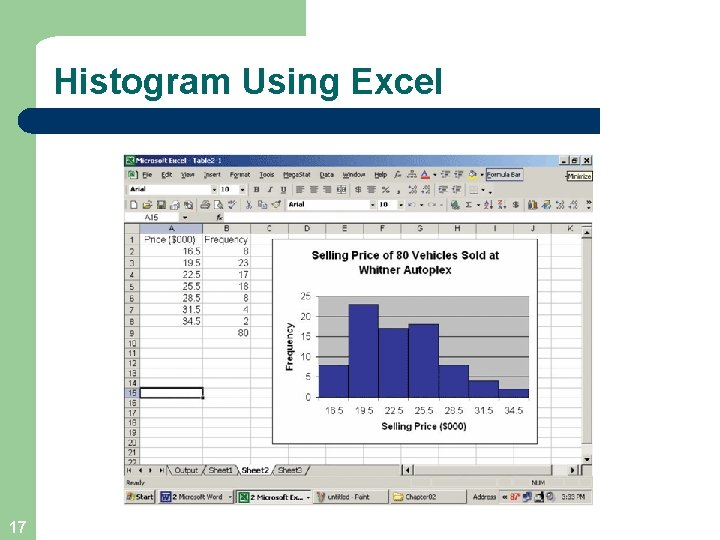Histogram Using Excel 17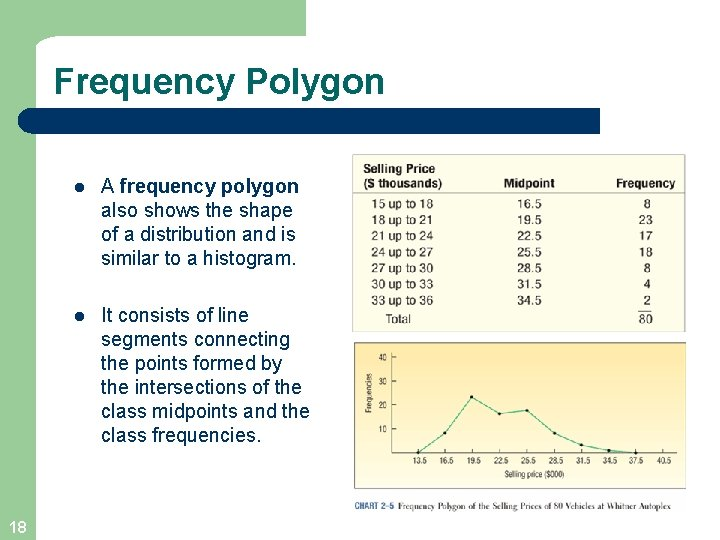Frequency Polygon 18 l A frequency polygon also shows the shape of a distribution and is similar to a histogram. l It consists of line segments connecting the points formed by the intersections of the class midpoints and the class frequencies.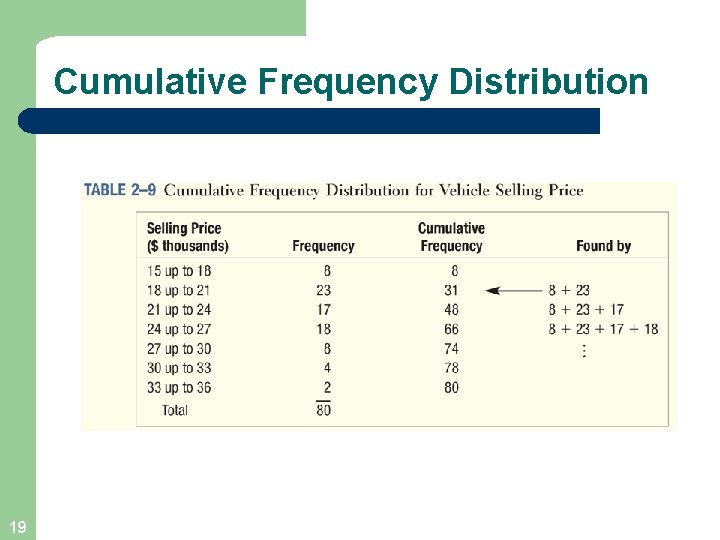Cumulative Frequency Distribution 19Cumulative Frequency Distribution 20End of Chapter 2 21